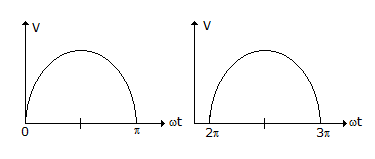# Electronics and Communication Engineering - Power Electronics

### Exercise :: Power Electronics - Section 8

46.

Assertion (A): Thyristors can be used in controlled heating, excitation systems of alternators, speed control of motors and HVDC

Reason (R): A static var system using thyristors is very commonly used in high voltage ac systems.

 A. Both A and R are correct and R is correct explanation of A B. Both A and R correct but R is not correct explanation of A C. A is correct but R is wrong D. A is wrong but R is correct

Explanation:

No answer description available for this question. Let us discuss.

47.

In a dual converter operated in circulating current mode, the circulating current flows

 A. when load current is zero B. when load current is non-zero C. even when load current is zero D. none of the above

Explanation:

No answer description available for this question. Let us discuss.

48.

For a single phase half wave converter feeding a resistive load, the firing angle a. The waveshape in below figure is forA. output voltage B. input voltage C. voltage drop across thyristor D. none of the above

Explanation:

No answer description available for this question. Let us discuss.

49.

A class B chopper operates in third quadrant.

 A. True B. False

Explanation:

No answer description available for this question. Let us discuss.

50.

In a full bridge circuit supplying an RLC under damped circuit

 A. the waveshapes of output voltage and load current are square B. the waveshapes of output voltage and load current are sinusoidal C. the waveshapes of output voltage is square but the waveshape of load current is nearly sinusoidal D. none of the above